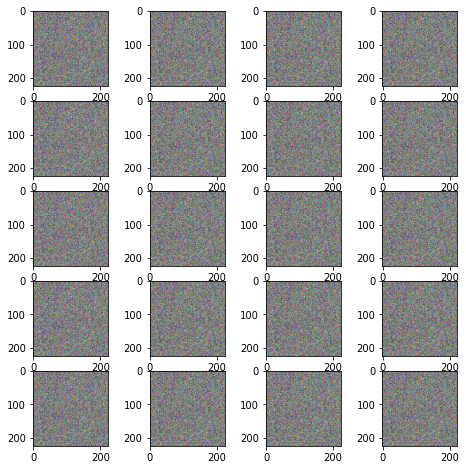# How to create image using same image multiple times?

``````import numpy as np
import matplotlib.pyplot as plt
import cv2 as cv

w = 10
h = 10

fig = plt.figure(figsize=(8, 8))
columns = 4
rows = 5
for i in range(1, columns*rows +1):
img = np.random.randint(10, size=(h,w))
plt.imshow(figure)
plt.show(
``````

I used this code but with this code I create the several images.
I want to create the image using same image using multiple time.
ex. take 1 image and use 25 time like 5*5 to create one image.

I’m not sure what the exact issue is, as a single figure is created with 20 subplots:In any case, this question doesn’t seem to be PyTorch-related, so you might get faster and better help on e.g. StackOverflow.

@ptrblck Thank yo for your reply. actually I send this question on StackOverflow as well.@ptrblck
I want to create my output image something like this.
I use one Image to create another image like this.
This is also one image but in 9 different patch.